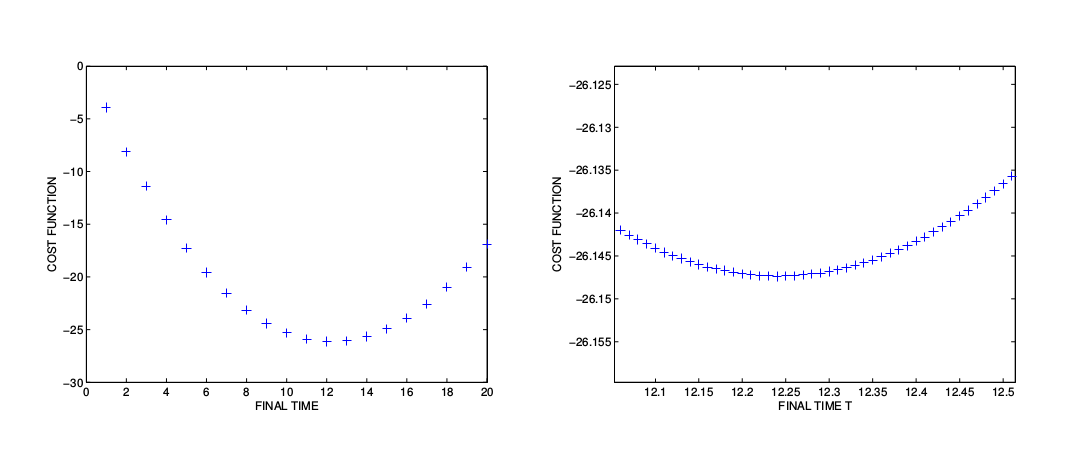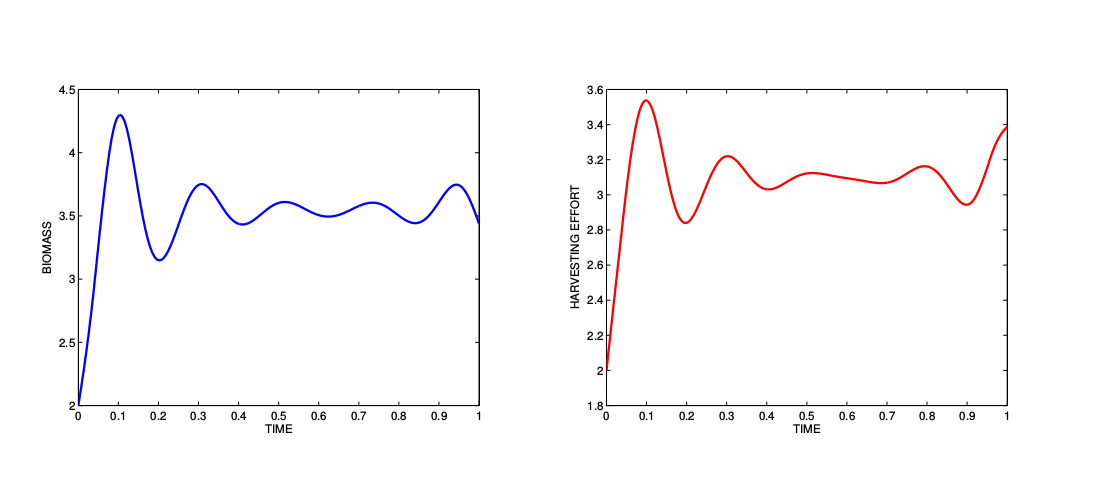# Harvest

We study here the delay problem studied in  and , originating from . The aim is to find the optimal harvesting of a renewable ressource whose growth follows a logistic function. Denoting$Latex formula$ the biomass of population and$Latex formula$ the harvesting effort, the optimal control problem is stated as$Latex formula$

with the harvesting cost$Latex formula$, the growth rates$Latex formula$, the discount rate$Latex formula$ and market price$Latex formula$, and the growth delay$Latex formula$. Bocop can handle the delayed term$Latex formula$ without having to perform the classical Guinn transformation (), but for a fixed final time only. Therefore we perform a batch of optimizations for$Latex formula$, and iterate the process for$Latex formula$ to find a better estimate of the optimal time. Batch optimizations indicate an optimal final time$Latex formula$ with an objective$Latex formula$.Fig1: Delay problem. Cost function with respect to final time T.Fig2: Delay problem. Optimal state and control for T = 12.24.

References
 A. Boccia, P. Falugi, H. Maurer, and R. Vinter. Free time optimal control problems with time delays. pages 520–525. IEEE, 2013.
 L. Goellmann, D. Kern, and H. Maurer. Optimal control problems with delays in state and control variables subject to mixed control-state constraints. Optimal Control Applications and Methods, 30(4):341–365, 2009.
 T. Guinn. Reduction of delayed optimal control problems to non-delayed problems. Journal of Optimization Theory and Applications, 18(3):371–377, 1976.
 R. May. Stability and Complexity in Model Ecosystems. 1975.